Subsections

# Self-Consistent Field Theory

## Inhomogeneous systems

In the previous lecture we obtained for Ising lattice: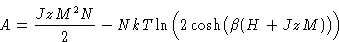(1)
This is for homogeneous systems. Now M depends on x. We introduce layers normal to x axis: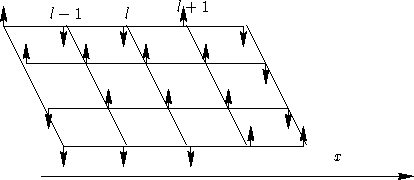and M becomes M(l).

Consider a simple cubic lattice. Each spin in the layer l has z=6 neighbors:

• One neighbor in the layer l+1
• One neighbor in the layer l-1
• z-2 neighbors in the layer l

Changes to equation (1): substitute N by sum over layers. Then:

1.
The first term describes mean interaction of a given spin with z neighbors. Substitute zM2 for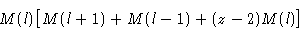2.
The second term describes molecular field acting on a given spin (in the layer l). Substitute zM by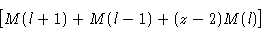Assumption:
Magnetization M(l) changes at distances much larger than the lattice size a0
This means that the difference between neighboring layers is small: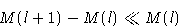We substitute M(l) by a smooth function M(x) and use the expansion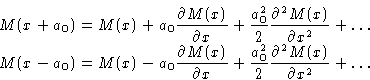and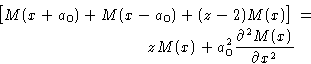## Conclusion

We see that in SCF A can be represented as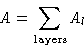with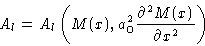We will not solve the resulting non-linear equations, but rather use this in the Landau theory.Next: Landau Theory Up: Spatial Inhomogeneity. Interfaces Previous: Problem

© 1997 Boris Veytsman and Michael Kotelyanskii
Thu Oct 16 20:58:44 EDT 1997